Optimizing a Data Center Using Integer Programming
posted at March 2, 2016 with tags algorithm

Optimize a Data Center is a challenging programming problem presented in the Qualification Round of the Hash Code 2015. Among 230 teams, What’s in a name? from École normale supérieure ranked first in the qualification, but third in the final round. By challenging, I mean it is not possible to come up with a deterministic polynomial-time optimal answer. I am not in a position to either provide a rigorous proof of its complexity or its reduction to a known NP-hard problem. But in this blog post I will investigate the following question: Can we provide an optimal solution using integer programming? In practice, that would allow us to come up with an optimal solution to small-sized problems. Without further ado, let’s start with the problem definition.

# The Problem

Here, I will present a brief summary of the actual problem. A data center is modeled as rows​ of slots ​in which servers can be placed. And some of the slots are known to be unavailable.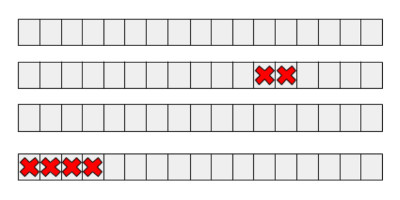Each server is characterized by its size and capacity​. Size is the number of consecutive slots occupied by the machine. Capacity is the total amount of CPU resources of the machine (an integer value).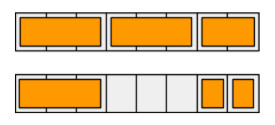Servers in a data center are also logically divided into pools. ​Each server belongs to exactly one pool. The capacity of a pool is the sum of the capacities of the available ​servers in that pool.

The guaranteed capacity ​of a pool is the minimum capacity it will have when at most one data center row goes down. Given a schema of a data center and a list of available servers, the goal is to assign servers to slots within the rows​ and to logical pools ​so that the lowest guaranteed capacity​ of all pools is maximized.

Consider the following data center schema and a list of available servers. For simplicity, it is assumed that server capacities are equal to server sizes.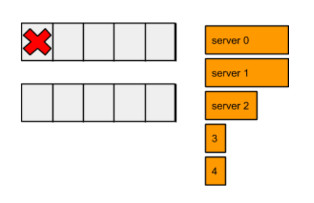Following layout is a solution to the above given problem. Here different pools are denoted in distinct colors.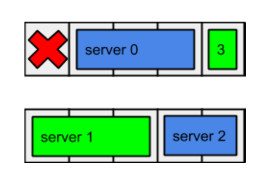# Preliminaries

Before modeling the IP (Integer Program), I will start with stating the problem input.

• $R$ ​denotes the number of rows in the data center
• $S$ denotes the number of slots in each row of the data center
• $U \leq RS$ denotes the number of unavailable slots
• $P$ denotes the number of pools to be created
• $M \leq RS$ denotes the number of servers to be allocated
• $z_k$ and $c_k$ denote $k$th server’s respectively size and capacity
• $r_i$ and $s_i$ denote $i$th unavailable slot’s respectively row and slot indices

While modeling the formulation, I will need to provide constraints to avoid placing servers to unavailable slots. Rather than doing that, Atabey Kaygun hinted me to represent the data in blocks. That is, instead of $U$, $r_i$, and $s_i$, I will transform this data into a single lookup table called $z(i, j)$ that denotes the size of the available slots at $i$th row and $j$th block. For instance, consider the following layout: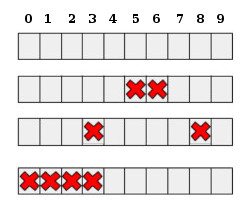Here blocks will look as follows:

# The Integer Programming Model

For simplicity, I will adopt the following index notation:

• $i$ denotes row indices ($0 \leq i \leq R$)
• $j$ denotes block (not slot!) indices (varies per row)
• $k$ denotes server indices ($0 \leq k \leq M$)
• $\ell$ denotes pool indices ($0 \leq \ell \leq P$)

Given that $z(i, j)$ denotes the available blocks, the IP model can be defined as follows:

# Avoiding Minimax Constraint

The presented IP model contains a minimax objective, which to the best of my knowledge is not tractable by popular linear programming optimizers, such as CPLEX or lpsolve. But I have a trick in my pocket to tackle that. Let’s assume that we know the optimal objective, say $g^*$. Then we can model the entire IP as follows:

What this model states is this: I am not interested in the optimization objective, return me the first found feasible solution. That is, the optimizer will return us the first $a(i, j, k, \ell)$ variable set the moment it finds a feasible solution satisfying $g(\ell, i) \geq g^*, \, \forall \ell, i$ constraints.

Now things are getting interesting. If we can find bounds to $g^*$, than we can use these bounds to bisect the optimal $g^*$! For the lower bound, we know that $g_i = 0 \leq g^*$. The upper bound is a little bit tricky, but we can come up with a quite loose bound: $g_f = \frac{1}{P} \sum_k c_k \gg g^*$. (I will not go into details of how to come up with a stricter upper bound.) So by picking $g^* \in (g_i, g_f)$ we can bisect the optimal guaranteed capacity.

# The Solver

For testing purposes, I wrote a simple Python script that reads an input problem file and calls CPLEX iteratively. A sample output of the script is as follows:

\$ ./dc.py ./cplex.sh prob/dc-min.in soln/dc-min 0
2016-03-03 08:35:25 DEBUG    reading setup: prob/dc-min.in
2016-03-03 08:35:25 DEBUG    R=2, S=5, U=1, P=2, M=5
2016-03-03 08:35:25 INFO     solving for 0 <= g=8 < 16
2016-03-03 08:35:25 DEBUG    writing problem: soln/dc-min-8.lp
2016-03-03 08:35:25 DEBUG    running solver
2016-03-03 08:35:25 DEBUG    writing cplex output: soln/dc-min-8.out
2016-03-03 08:35:25 DEBUG    no solution
2016-03-03 08:35:25 DEBUG    stepping back
2016-03-03 08:35:25 INFO     solving for 0 <= g=4 < 8
2016-03-03 08:35:25 DEBUG    writing problem: soln/dc-min-4.lp
2016-03-03 08:35:25 DEBUG    running solver
2016-03-03 08:35:25 DEBUG    writing cplex output: soln/dc-min-4.out
2016-03-03 08:35:25 DEBUG    solution score: 5
2016-03-03 08:35:25 DEBUG    writing solution: soln/dc-min-4.soln
2016-03-03 08:35:25 DEBUG    stepping forward
2016-03-03 08:35:25 INFO     solving for 5 <= g=6 < 8
2016-03-03 08:35:25 DEBUG    writing problem: soln/dc-min-6.lp
2016-03-03 08:35:25 DEBUG    running solver
2016-03-03 08:35:25 DEBUG    writing cplex output: soln/dc-min-6.out
2016-03-03 08:35:25 DEBUG    no solution
2016-03-03 08:35:25 DEBUG    stepping back
2016-03-03 08:35:25 INFO     solving for 5 <= g=5 < 6
2016-03-03 08:35:25 DEBUG    writing problem: soln/dc-min-5.lp
2016-03-03 08:35:25 DEBUG    running solver
2016-03-03 08:35:25 DEBUG    writing cplex output: soln/dc-min-5.out
2016-03-03 08:35:25 DEBUG    solution score: 5
2016-03-03 08:35:25 DEBUG    writing solution: soln/dc-min-5.soln
2016-03-03 08:35:25 DEBUG    stepping forward


It also outputs intermediate IP formulation files, CPLEX output, and solution file. The format of the problem and solution files are detailed in the official problem description.